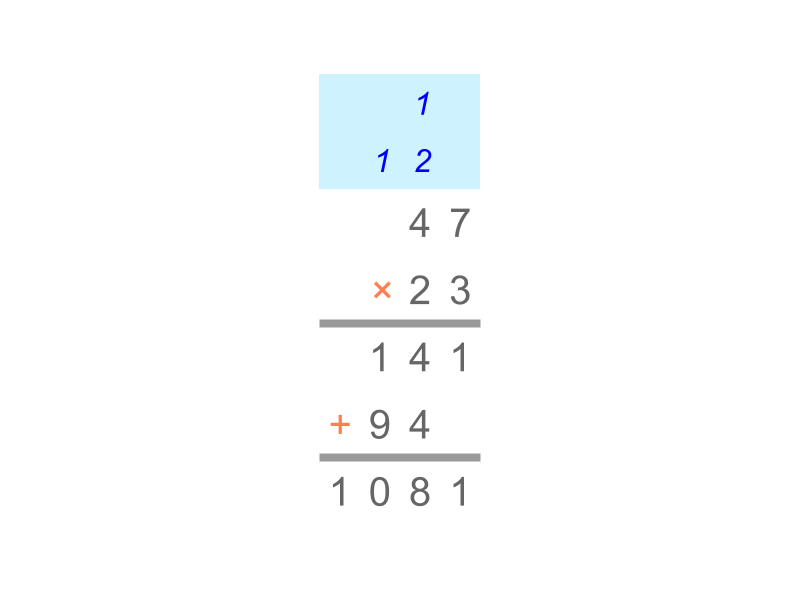maths > integers

Integer Multiplication: Simplified Procedure

what you'll learn...

overview

The multiplication in first principles was explained as repetition of integer multiplicand, the integer multiplier number of times. This establishes the simplified procedure : Sign-Property of Integer Multiplication.

regroupingIn whole numbers, we had studied the following.

Multiplication by Place-value with Regrouping - Simplified Procedure : Two numbers are multiplied as illustrated in the figure.
Note: The procedure combines 10$10$ units into 1$1$ ten as carry over and so on for higher place-values.

The product of whole numbers 4×3=12$4 \times 3 = 12$ is understood in integers as:

received:4$\textrm{\left(r e c e i v e d\right\rangle} 4$ is repeatedly put in 3$3$ times.

The product of integers 4×3=12$- 4 \times 3 = - 12$ is understood as $\textrm{\left(g i v e n\right\rangle} 4$ is repeatedly put in $3$ times.

The product of integers $4 \times - 3 = - 12$ is understood as $\textrm{\left(r e c e i v e d\right\rangle} 4$ is repeatedly taken away $3$ times.

The product of integers $- 4 \times - 3 = + 12$ is understood as $\textrm{\left(g i v e n\right\rangle} 4$ is repeatedly taken away $3$ times.

simplify

Summary of integer multiplication illustrative examples:

•  $4 \times 3 = 12$
$\textrm{\left(r e c e i v e d\right\rangle} 4$ is put-in $3$ times

•  $\left(- 4\right) \times 3 = - 12$
$\textrm{\left(g i v e n\right\rangle} 4$ is put-in $3$ times

•  $4 \times \left(- 3\right) = - 12$
$\textrm{\left(r e c e i v e d\right\rangle} 4$ is taken-away $3$ times

•  $\left(- 4\right) \times \left(- 3\right) = 12$
$\textrm{\left(g i v e n\right\rangle} 4$ is taken-away $3$ times

Based on this, the multiplication is simplified as

•  +ve $\times$ +ve $=$ +ve

•  -ve $\times$ +ve $=$ -ve

•  +ve $\times$ -ve $=$ -ve

•  -ve $\times$ -ve $=$ +ve

examples

Find the product of the multiplication $22 \times \left(- 1\right)$
The answer is "$- 22$"

Find the product of the multiplication $0 \times \left(- 32\right)$
The answer is "both the above"

Find the product of the multiplication $- 222 \times \left(- 88\right)$
The answer is "a positive number".

summary

Integer Multiplication -- Simplified Procedure : The sign of the product is decided by signs of multiplicand and multiplier as follows.

Sign-Property of Integer Multiplication
•  positive $\times$ positive $=$ positive
•  positive $\times$ negative $=$ negative
•  negative $\times$ positive $=$ negative
•  negative $\times$ negative $=$ positive

The absolute value of the product is calculated by whole number multiplication of absolute values of the multiplicand and multiplier.

Outline